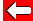# Averages: Range

Another useful statistical measure involved with averages is range. This is simply how wide the numbers fall, and is found by subtracting the smallest number from the largest number.

e.g. What was the range of our darts player's scores: 80, 100, 62, 180, 21, 58

Range = biggest number - smallest number
= 180 - 21
= 159Go back a pageMaths MenuGo to next page